# Lecture 8: Summarizing Data Part 2: Plots

## Recap

• Summary statistics are ways of describing the distribution of a variable
• Examples include mean, percentiles, SD, and specific percentiles of interest (median, min, max)
• Another, very good way to describe a variable is by actually showing that distribution with a graph
• I showed you plenty of graphs last time, this time I’m going to show you how to make them yourself!

• As I mentioned before, we’re going straightforward, doing our plotting with base R
• In the case of plotting, it’s going to be easier to use base R, but also not look as good as the alternative, `ggplot2`, which is also in the tidyverse package.
• `ggplot2` code for plots will be available in the slides. Just search for ‘ggplot’
• And have also been for most previous slides - I’ve been making most graphs with it!
• Don’t forget extra credit opportunity for learning `ggplot2`

## Plot types we will cover:

• Density plots (for continuous)
• Histograms (for continuous)
• Box plots (for continuous)
• Bar plots (for categorical)
• Plus:
• Putting lines on plots
• Makin ’em look good!

## Plots we won’t:

• Lots and lots and lots of plot options
• Mosaics, Sankey plots, pie graphs
• Some aren’t common in Econ but could be!
• Others are just too tricky (like maps)
• See The R Graph Gallery

## Density plots and histograms

• Density plots and histograms will show you the full distribution of the variable
• Values along the x-axis, and how often those values show up on y
• The difference - density plots will present a smooth line by averaging nearby values
• A histogram will create “bins” and tell you how many observations fall into each
• (don’t forget we can save plots using Export in the Plots pane)

## Density plots

• To make a density plot, we take our variable and make a `density()` object out of it, then `plot()` that thing!
• Let’s play around with data from the `Ecdat` package
``````install.packages('Ecdat')
library(Ecdat)
data(MCAS)
plot(density(MCAS\$totsc4))``````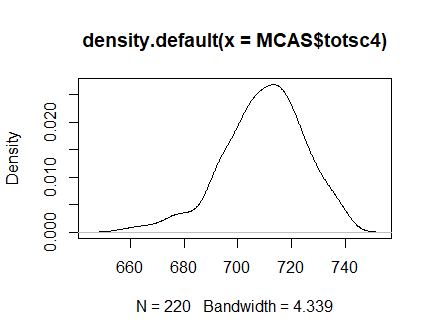## Titles and labels

• Readability is super important in graphs
• Add labels and titles! Titles with ‘main’ and axis labels with ‘xlab’ and ‘ylab’
``````plot(density(MCAS\$totsc4),main='Massachusetts Test Scores',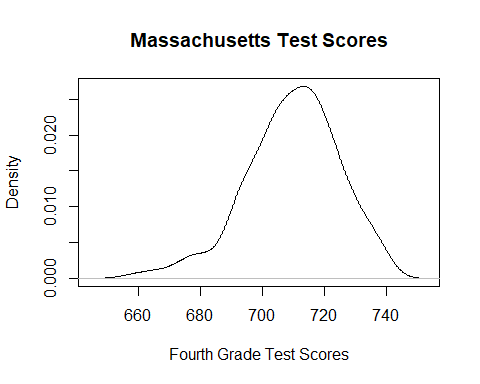## Histograms

• Histograms require a little less typing - just `hist()`
``hist(MCAS\$totsc4)``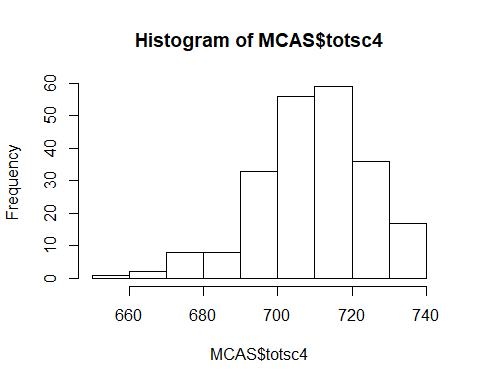## Histograms

• These need labels too! Other important options:
• Do proportions with `freq=FALSE`, or change how many bins there are, or where they are, with `breaks`
``````hist(MCAS\$totsc4,xlab="Fourth Grade Test Scores",
main="Test Score Histogram",freq=FALSE,breaks=50)``````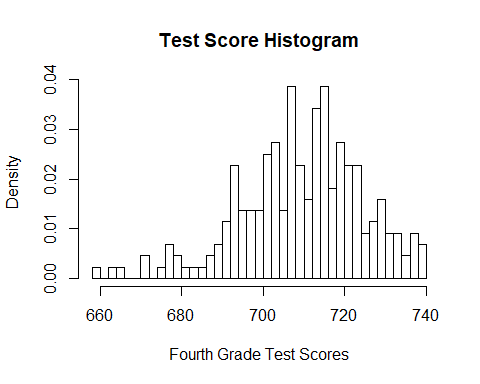## Box plots

• Less common in economics, but a good way to look at your data
• And check for outliers!
• Basically a summary table in graph form
• Shows the 25%, 50%, 75% percentiles
• Plus lines that represent 1.5 IQR (75th minus 25th)
• And dots for anything otuside that range

## Box plots

• Simple!
• Note the negative outliers - not necessarily a problem but good to know!
``boxplot(MCAS\$totsc4,main="Box Plot of 4th Grade Scores")``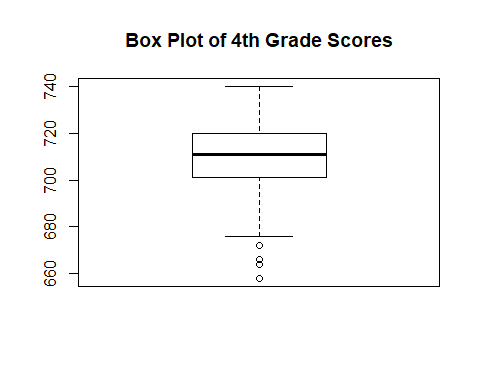## Box plots

• Easy to look at multiple variables at once, too
``boxplot(select(MCAS,totsc4,totsc8),main="Box Plot of 4th Grade Scores")``